# GMAT Math : Equilateral Triangles

## Example Questions

1 3 Next →

### Example Question #1 : Calculating The Perimeter Of An Equilateral Triangle

Given the following regarding Triangle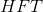.

I)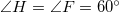II) Sideis equal tolight-years

What is the length of side?

Possible Answers:light years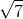light yearslight yearslight yearslight years

Correct answer:light years

Explanation:

The trick to this one is to carefully put together what you are given.

We know that two of our angles are equal to 60 degrees. This means that the last angle is also 60 degrees. This make HFT an equilateral triangle.

Equilateral triangles always have equal sides and equal angles, so our last side has to be 7 light years as well.

### Example Question #2 : Calculating The Perimeter Of An Equilateral Triangle

Calculate the perimeter of an equilateral triangle whose side length is.

Possible Answers:Correct answer:Explanation:

The perimeter of an equilateral triangle is: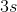Thus.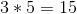### Example Question #3 : Calculating The Perimeter Of An Equilateral Triangle

Find the perimeter of an equilateral triangle whose side length is.

Possible Answers:Correct answer:Explanation:

To find the perimeter, you must multiply the side length by. Thus,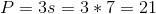### Example Question #4 : Calculating The Perimeter Of An Equilateral Triangle

Calculate the perimeter of an equilateral triangle whose side length is.

Possible Answers:Correct answer:Explanation:

To solve, simply multiply the side length by. Thus,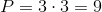1 3 Next →

### All GMAT Math Resources